Examples using MPI_SCATTER, MPI_SCATTERV

## Examples using MPI_SCATTER, MPI_SCATTERV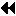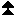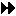Up: Scatter Next: Gather-to-all Previous: Scatter

```

The reverse of Example Examples using  MPI_GATHER,  MPI_GATHERV
.
Scatter sets of 100 ints from the root to each process in the group.
See figure 8
.

MPI_Comm comm;
int gsize,*sendbuf;
int root, rbuf;
...
MPI_Comm_size( comm, &gsize);
sendbuf = (int *)malloc(gsize*100*sizeof(int));
...
MPI_Scatter( sendbuf, 100, MPI_INT, rbuf, 100, MPI_INT, root, comm);

```
figure=mycoll-fig7.ps,width=3.50in
Figure 8: The root process scatters sets of 100  ints to each process in the group.

```

The reverse of Example Examples using  MPI_GATHER,  MPI_GATHERV
.
The root process scatters sets of 100 ints to the other processes,
but the sets of 100 are  stride ints apart in the sending buffer.
Requires use of  MPI_SCATTERV.
Assume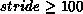.  See figure 9
.

MPI_Comm comm;
int gsize,*sendbuf;
int root, rbuf, i, *displs, *scounts;

...

MPI_Comm_size( comm, &gsize);
sendbuf = (int *)malloc(gsize*stride*sizeof(int));
...
displs = (int *)malloc(gsize*sizeof(int));
scounts = (int *)malloc(gsize*sizeof(int));
for (i=0; i<gsize; ++i) {
displs[i] = i*stride;
scounts[i] = 100;
}
MPI_Scatterv( sendbuf, scounts, displs, MPI_INT, rbuf, 100, MPI_INT,
root, comm);

```
figure=mycoll-fig8.ps,width=3.50in
Figure 9: The root process scatters sets of 100  ints, moving by  stride ints from send to send in the scatter.

```

The reverse of Example Examples using  MPI_GATHER,  MPI_GATHERV
.
We have a varying stride between blocks at sending (root) side,
at the receiving side we receive into the ith column of a 100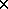150
C array.
See figure 10
.

MPI_Comm comm;
int gsize,recvarray,*rptr;
int root, *sendbuf, myrank, bufsize, *stride;
MPI_Datatype rtype;
int i, *displs, *scounts, offset;
...
MPI_Comm_size( comm, &gsize);
MPI_Comm_rank( comm, &myrank );

stride = (int *)malloc(gsize*sizeof(int));
...
/* stride[i] for i = 0 to gsize-1 is set somehow
* sendbuf comes from elsewhere
*/
...
displs = (int *)malloc(gsize*sizeof(int));
scounts = (int *)malloc(gsize*sizeof(int));
offset = 0;
for (i=0; i<gsize; ++i) {
displs[i] = offset;
offset += stride[i];
scounts[i] = 100 - i;
}
/* Create datatype for the column we are receiving
*/
MPI_Type_vector( 100-myrank, 1, 150, MPI_INT, &rtype);
MPI_Type_commit( &rtype );
rptr = &recvarray[myrank];
MPI_Scatterv( sendbuf, scounts, displs, MPI_INT, rptr, 1, rtype,
root, comm);

```
figure=mycoll-fig9.ps,width=4.00in
Figure 10: The root scatters blocks of 100-i ints into column  i of a 100\$ x \$150 C array. At the sending side, the blocks are  stride[i] ints apart.Up: Scatter Next: Gather-to-all Previous: Scatter